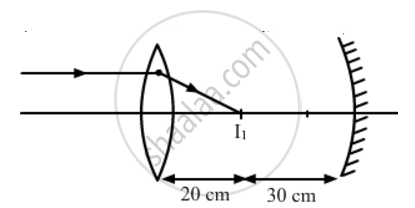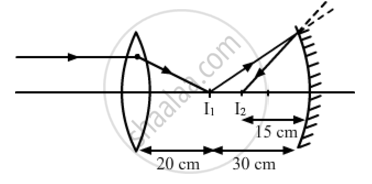# A Convex Lens of Focal Length 20cm is Placed Coaxially with a Concave Mirror of Focal Length 10 cm at a Distance of 50 cm Apart from Each Other. a Beam of Light Coming Parallel to the Principal Axis is Incident on the Convex Lens. - Physics

A convex lens of focal length 20 cm is placed coaxially with a concave mirror of focal length 10 cm at a distance of 50 cm apart from each other. A beam of light coming parallel to the principal axis is incident on the convex lens. Find the position of the final image formed by this combination. Draw the ray diagram showing the formation of the image

#### Solution

Let us first locate the image of the point object S formed by the convex lens.

Here:

u = -∞ cm and f = 20 cm

From the lens formula, we have:

1/v-1/u=1/f

=>1/v=1/f+1/u

=>1/v=1/20+1/(-oo)

=>1/v=1/20

⇒ 20 cm

The positive sign shows that the image is formed to the right of the lens, as shown in the following figure.The image I1 is formed in front of the mirror and hence, acts as a real source for the mirror. The concave mirror forms the image I2, whose distance from the mirror can be calculated as:

1/v=1/u=1/f

Here:

u=-30 cm ; f=-10 cm

=>1/v=1/f-1/u

=>1/v=-1/10+1/30

=>1/v=(1-3)/30=(-2)/30

⇒ − 15 cm

Hence, the final image is formed at a distance of 15 cm from the concave mirror, as shown in the following figure.Concept: Concave Mirror
Is there an error in this question or solution?
2013-2014 (March) All India Set 2

Share